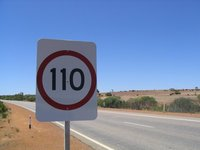# Speed Conversions

Alignments to Content Standards: 6.RP.A.3.d

Jessica sees the following speed limit sign while visiting Australia where the units for speed are kilometers per hour:1. A conversion table indicates that 1 mile is 1.6 km. With this conversion rate, is the speed limit greater than or less than 65 mph? Explain.
2. Jessica finds out that 1 mile is not exactly 1.6 km. This number has been rounded to the nearest tenth. Does this influence the answer to part (a)?

## IM Commentary

The goal of this task is to perform a unit conversion in the context of speed while also focusing on the precision of the conversion factor. Because the conversion rate is a decimal, this task should be used after students have gained some familiarity with ratio and rate reasoning. The decimal has only been carried out to the tenths place to make the calculation more accessible. Many conversions between metric units and standard units are inexact and so the accuracy issue comes up repeatedly. This can always be handled by using more decimals in the conversion factor, increasing the accuracy. On the other hand, there is a trade-off because the calculations become more complex when the conversion factor is more exact. For the second part of this task, the numbers are more challenging and the teacher will have to decide whether or not to allow technology to complete this part of the task: to the extent that the main intention here is to reason about rounding numbers and the impact this has on the final result, use of technology might be appropriate.

In addition to converting 65 miles to kilometers (or 110 kilometers to miles), students might also approach this task via a ratio table or estimation. These two approaches are valuable both for building an understanding of how unit conversions give rise to ratios and for developing computational fluency. The mental calculation approach is challenging because the two rates are relatively close and so a relatively high degree of accuracy must be maintained.

## Solutions

Solution: Rate Conversion

1. The speed limit is 110 $\frac{\text{km}}{\text{hour}}$ and there are 1.6 kilometers in a mile. This means that there are 110 $\div$ 1.6 miles per hour: $$110 \frac{\text{km}}{\text{hour}} \div 1.6 \frac{\text{km}}{\text{mile}} = 110 \div 1.6 \frac{\text{miles}}{\text{hour}}.$$ We can find 110 $\div$ 1.6 using long division and it is 68.75. So the speed limit in Australia is higher than 65 mph.
2. If the conversion rate of 1.6 km per mile has been rounded off to the nearest tenth. Then the number of kilometers per mile is at least 1.55 and less than 1.65. We find 110 $\div$ 1.55 $\approx$ 71 and 110 $\div$ 1.65 $\approx$ 67. Both values have been rounded to the nearest whole number. So no matter how many kilometers are in a mile (between 1.55 and 1.65) the speed limit of 110 kilometers per hour in Australia is higher than 65 miles per hour.

Solution: Ratio Table for part (a)

We can solve this problem with a ratio table. The first line shows the given information that one mile is equivalent to 1.6 km:

Kilometers Miles
1.6 1
16 10
80 50
96 60
112 70

Since 112 kilometers is 70 miles, 110 kilometers is a little less than 69 miles and so 110 kilometers per hour is more than 65 miles per hour. We could extend the table to find the exact conversion for 110 kilometers:

Kilometers Miles
1.6 1
16 10
80 50
96 60
112 70
110.4 69
110 68.75

The last line uses the fact that since there are 1.6 kilometers in a mile there are 0.4 kilometers in $\frac{1}{4}$ of a mile.

A ratio table can be used for the conversions in part (b), but the numbers are more complex which makes this a more challenging approach. A double number line could be an appropriate representation but the large numbers would make it difficult to maintain an accurate scale. Therefore, the ratio table is a more appropriate technique for this task.

Solution: Estimation

1. In 65 miles there will be 65 $\times$ 1.6 kilometers so we need to compare 65 $\times$ 1.6 to 110. One way to do this is to find 65 $\times$ 16 and then divide by 10. Multiplying by 16 can be accomplished mentally by successively doubling 4 times. If we double 65 we get 130. Continuing to double gives 260, 520, and 1040. Now we divide by 10 to get 104. In 65 miles there are 104 kilometers so 110 kilometers an hour is faster than 65 miles per hour.
2. Here the key calculation will be 65 $\times$ 1.65 since the number of kilometers in a mile is less than 1.65 if the number 1.6 has been rounded to the nearest tenth. Using the distributive property, we have \begin{align}65 \times 1.65 &= 65 \times (1.6 + 0.05)\\ &= 65 \times 1.6 + 65 \times 0.05\\ &= 104 + 0.05 \times 65.\end{align} One hundredth of 65 is 0.65 so five hundredths of 65 is a little more than 3. So there are at most a little more than 107 kilometers in 65 miles if the conversion rate of 1.6 kilometers per hour is rounded to the nearest tenth. Again, the speed limit in Australia is more than 65 miles per hour.